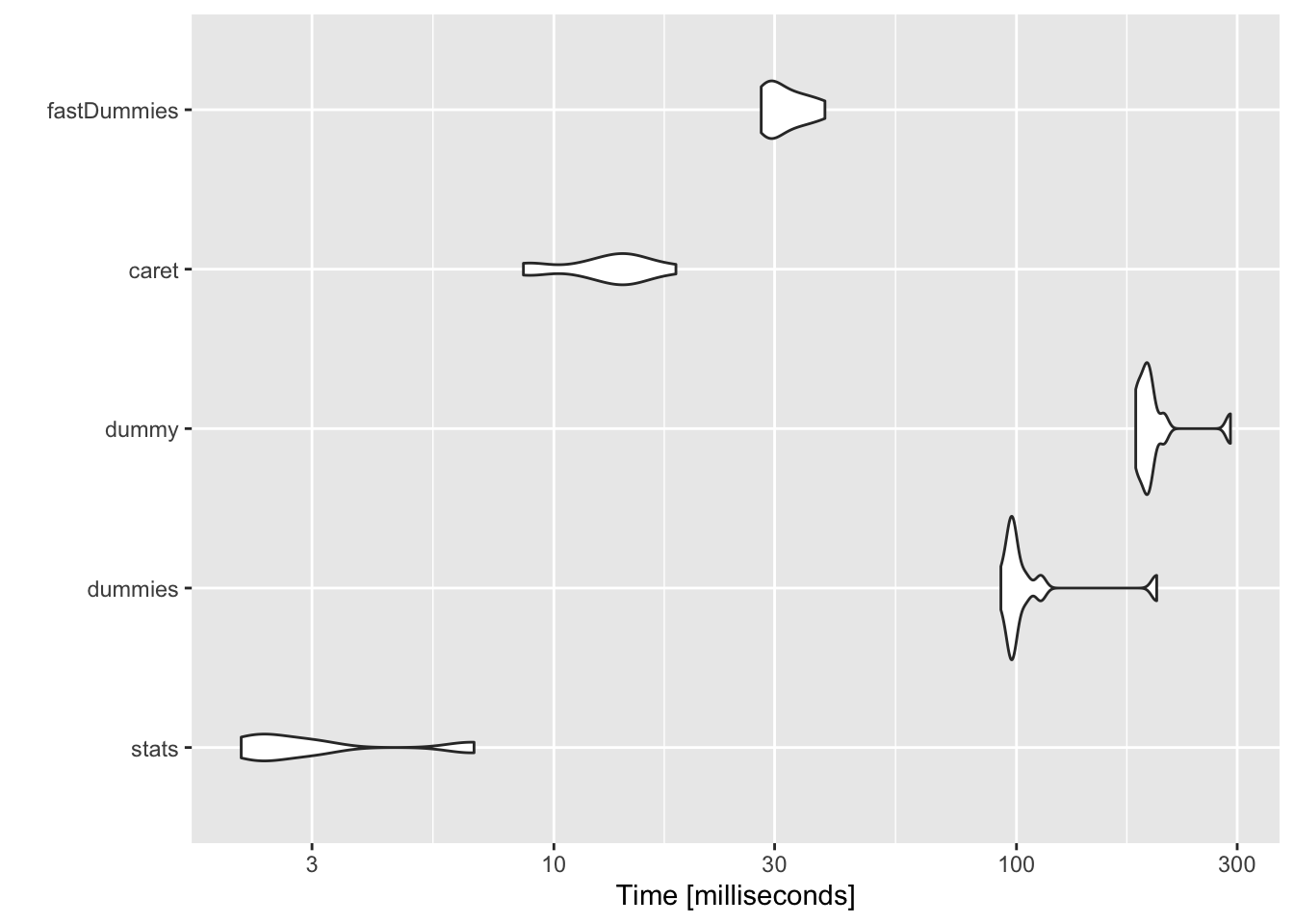# Perf Benchmarking Dummy Variables - Part II

Benchmarking
Is `{fastDummies}` any better than `{stats}` to create dummy variables? Let’s find out.
Author

Rahul

Published

December 16, 2020## tl;dr

• `{stats}` continues to dominate the speed tests
• `{fastDummies}` had similar speeds only for dataframes with rows ~1M
• `{dummy}` and `{dummies}` are the slowest

## Motivation

In 2017, I compared the performance of four packages `{stats}`, `{dummies}`, `{dummy}` and `{caret}` to create dummy variables in this post.

Jacob Kaplan of UPenn has created a new package `{fastdummies}` which claims to be faster than other existing packages.

Let’s test it out.

## Machine

I’m running these tests on a 2019 MacBook Pro running macOS Catalina (10.15.7) on a 2.4 GHz 8-Core Intel i9 with 32 MB 2400 MHz DDR4, in a docker container running:

``````platform       x86_64-pc-linux-gnu
arch           x86_64
os             linux-gnu
system         x86_64, linux-gnu
status
major          4
minor          0.0
year           2020
month          04
day            24
svn rev        78286
language       R
version.string R version 4.0.0 (2020-04-24)
nickname       Arbor Day ``````

## Perf Testing

### A quick test

Create a test dataset…

``````NROW  <- 1e4
fac_levels <- c(4, 4, 5, 5, 7, 7, 9, 9)
input_data <- tibble::tibble(
facVar_1 = as.factor(sample(LETTERS[1:fac_levels], size = NROW, replace = TRUE)),
facVar_2 = as.factor(sample(LETTERS[1:fac_levels], size = NROW, replace = TRUE)),
facVar_3 = as.factor(sample(LETTERS[1:fac_levels], size = NROW, replace = TRUE)),
facVar_4 = as.factor(sample(LETTERS[1:fac_levels], size = NROW, replace = TRUE)),
facVar_5 = as.factor(sample(LETTERS[1:fac_levels], size = NROW, replace = TRUE)),
facVar_6 = as.factor(sample(LETTERS[1:fac_levels], size = NROW, replace = TRUE)),
facVar_7 = as.factor(sample(LETTERS[1:fac_levels], size = NROW, replace = TRUE)),
facVar_8 = as.factor(sample(LETTERS[1:fac_levels], size = NROW, replace = TRUE))
)
str(input_data)``````
``````tibble [10,000 × 8] (S3: tbl_df/tbl/data.frame)
\$ facVar_1: Factor w/ 4 levels "A","B","C","D": 1 4 4 3 3 2 4 1 3 1 ...
\$ facVar_2: Factor w/ 4 levels "A","B","C","D": 4 1 4 4 4 4 4 3 3 4 ...
\$ facVar_3: Factor w/ 5 levels "A","B","C","D",..: 5 1 5 5 5 4 4 4 3 2 ...
\$ facVar_4: Factor w/ 5 levels "A","B","C","D",..: 2 3 1 1 5 5 1 4 2 3 ...
\$ facVar_5: Factor w/ 7 levels "A","B","C","D",..: 3 5 6 3 5 2 5 2 7 6 ...
\$ facVar_6: Factor w/ 7 levels "A","B","C","D",..: 5 2 1 3 3 5 5 6 2 2 ...
\$ facVar_7: Factor w/ 9 levels "A","B","C","D",..: 3 6 7 5 8 8 8 8 3 8 ...
\$ facVar_8: Factor w/ 9 levels "A","B","C","D",..: 5 8 7 3 6 2 7 8 8 2 ...``````

Run microbenchmark…

``````stats_fn <- function(dat) stats::model.matrix(~.-1,dat)
dummies_fn <- function(dat) dummies::dummy.data.frame(as.data.frame(dat))
dummy_fn <- function(dat) dummy::dummy(dat)
caret_fn <- function(dat) {caret::dummyVars(formula = ~.,data = dat) %>% predict(newdata = dat)}
fastDummies_fn <- function(dat) fastDummies::dummy_cols(dat)

microbenchmark::microbenchmark(
stats =       stats_fn(input_data),
dummies =     dummies_fn(input_data),
dummy =       dummy_fn(input_data),
caret =       caret_fn(input_data),
fastDummies = fastDummies_fn(input_data),
times = 10L
) %>% autoplot()```````stats` is still clearly the fastest of all the packages, for this moderately sized dataset.

### Dig a bit deeper

How does the performance vary when rows, columns, or number of factors are scaled?

First, make some functions to create dataframes with varying rows/cols/levels per variable, run benchmarks & extract median execution times.

``````make_data <- function(NROW = 10, NCOL = 5, NFAC = 5){
sapply(1:NCOL,
function(x) sample(LETTERS[1:NFAC],
size = NROW,
replace = TRUE)) %>%
as_tibble()

}
run_benchmark <- function(dat){
microbenchmark::microbenchmark(
stats =       stats_fn(dat),
dummies =     dummies_fn(dat),
dummy =       dummy_fn(dat),
caret =       caret_fn(dat),
fastDummies = fastDummies_fn(dat),
times = 10L
)
}
extract_median_time <- function(benchmarks){
as_tibble(benchmarks) %>%
dplyr::group_by(expr) %>%
summarize(median_ms = median(time) * 1e-6)
}``````

`make_data` makes a pretty simple tibble:

``make_data(NROW = 5, NCOL = 6, NFAC = 3)``
``````# A tibble: 5 × 6
V1    V2    V3    V4    V5    V6
<chr> <chr> <chr> <chr> <chr> <chr>
1 A     C     A     C     A     B
2 A     A     C     A     A     C
3 A     C     C     A     A     A
4 C     A     C     B     B     A
5 C     A     C     A     A     B    ``````

### How does performance scale by number of rows?

`stats` still rocks. With very large datasets, `fastDummies` approaches similar speed.

``````experiment_rows <- tibble::tibble(
nrows = 10^(1:6)
) %>%
dplyr::mutate(input_data = purrr::map(nrows, ~make_data(NROW = .x, NCOL = 5, NFAC = 5)),
benchmarks = purrr::map(input_data, ~run_benchmark(.x)),
median_times = purrr::map(benchmarks, ~extract_median_time(.x)))
experiment_rows %>%
dplyr::select(nrows, median_times) %>%
tidyr::unnest(cols = c(median_times)) %>%
dplyr::rename(Package = expr) %>%
tidyr::pivot_wider(names_from = Package, values_from = median_ms) %>%
dplyr::mutate(
dummies = dummies/stats,
dummy = dummy/stats,
caret = caret/stats,
fastDummies = fastDummies/stats,
stats = 1
) %>%
tidyr::pivot_longer(-nrows) %>%
ggplot(aes(nrows, value, color = name)) +
geom_line() +
geom_point(aes(text = glue::glue("<b>{title}</b> {verb} {y}x",
title = name,
verb = ifelse(name == "stats", ":", "slower by"),
y = ifelse(value > 2,
round(value),
round(value, digits = 1))))) +
scale_y_log10(labels = scales::label_number(accuracy = 1, suffix = "x")) +
scale_x_log10(breaks = 10^(1:6), labels = scales::label_number_si()) +
labs(x = "Number of Rows", y = "Relative Execution Rates",
title = "Row Performance (log-log scale)") -> p
ggplotly(p, tooltip = "text")``````

### How does performance scale by number of columns?

`stats` is the clear winner here.

### How does performance scale by number of levels?

Interestingly, number of levels per factor have little/no impact on performance for `stats`, `caret` and `dummies`. `fastDummies` & `dummies` show a positive correlation to levels.

See tl;dr